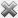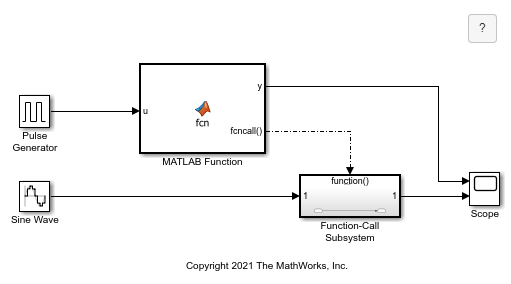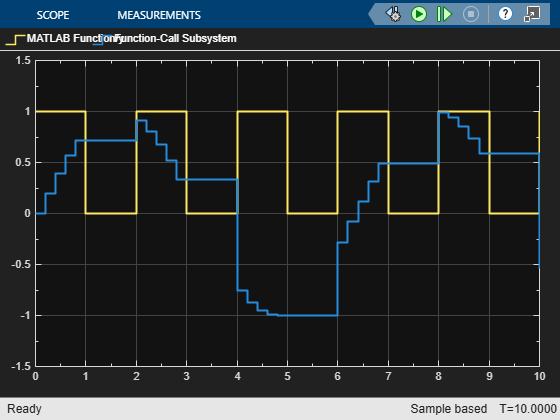## Manage Function Call Outputs of a MATLAB Function Block

A function call output is an event on the output port of a MATLAB Function block that causes a Function-Call Subsystem or Stateflow® chart in the model to execute. See Using Function-Call Subsystems. You can add function call outputs to MATLAB Function blocks and configure the function call outputs by using the Symbols pane and Property Inspector or the Model Explorer.

After creating a function call output, you need to call the function call output in the MATLAB Function block code.

### Create Function Call Outputs

To create function call outputs, use the Symbols pane or the Model Explorer.

To use the Symbols pane:

1. Open the MATLAB Function Block Editor. In the Function tab, click .

2. Click the Create Event buttonand select Add Function Call Output.

To delete the function call output, right-click the function call output name and select Delete.

To use the Model Explorer:

1. Open the Model Explorer. In the Modeling tab, in the Design section, click Model Explorer.

2. In the Model Hierarchy pane, select the MATLAB Function block.

3. Click Add > Function Call Output or the Add Function Call Output button.

To delete a function call output, select the function call output and click Edit > Delete or the Delete button.

### Enable a Function-Call Subsystem with a MATLAB Function Block

This example uses a MATLAB Function block with a function call output to trigger a subsystem.When the signal from the Pulse Generator block equals `1`, the MATLAB Function block executes the function call output. The block then continues to execute the function call output at each major time step. Double-click the MATLAB Function block to open the code.

```function y = fcn(u) ```
```y = u; if u == 1 fcncall() end ```

The function-call subsystem allows the signal from the Sine Wave block to propagate when the function-call triggers. When the function call is not triggered, the subsystem outputs the last propagated value. Run the model and open the Scope block to see the outputs.### Considerations When Triggering Function-Call Subsystems

If a MATLAB Function block triggers a function-call subsystem and outputs a signal to the same function-call subsystem, the signal to the function-call subsystem is delayed by one time step compared to the function call. At the moment of the function call output, the function-call subsystem sees the previous MATLAB Function block output port value even if the output data has been updated within the block MATLAB® code.

If Allow direct feedthrough is cleared, MATLAB Function blocks with function call outputs can cause unexpected results. When Allow direct feedthrough is disabled, the triggered subsystem receives the function call during model update, and therefore the function call output executes later than required. To avoid these issues, enable Allow direct feedthrough when using function call outputs. For more information, see Use Nondirect Feedthrough in a MATLAB Function Block.

### Set Function Call Output Properties

To adjust function call output properties, use the Symbols pane and Property Inspector or the Model Explorer.

To edit the properties by using the Symbols pane and Property Inspector, specify the function call output and open the Property Inspector by using of one of these approaches:

• In the Function tab, click . In the Symbols pane, select the function call output.

• In the Symbols pane, right-click the function call output name and click Inspect.

• In the Symbols pane, select the function call output. In the Modeling tab, in the Design Data section, click .

To edit the properties by using Model Explorer, click a function call output.

You can modify the following properties.

#### Name

Specifies the name of the function call output. Use the naming conventions used in MATLAB. You can only set this property in the Symbols pane or Model Explorer.

#### Port

Specifies the index of the port associated with the function call output. Function call output ports are numbered sequentially after output ports.

#### Description

Specifies the description of the function call output.